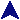Regular Expressions IRuby Example Code# Get a chomped string, or nil at EOF.
def getstr
print "Please enter a test string: "
str = gets
return str unless str
return str.chomp
end

# Test strings
while str = getstr
print "You entered: ", str, "\n"

# Run some random tests and print a descriptive message for ones which
# match.
num = 0
if str =~ /^\s*\$/ then
print "  > Your string is all blanks.\n"
next
end
if str =~ /Mommy/ then
print "  > Contains Mommy\n"
num += 1
end
print "  > Contains Mommy, then Daddy\n"
num += 1
end
if str !~ /CAT/ then
print "  > Does not contain CAT.\n"
num += 1
end
if str !~ /[Cc][Aa][Tt]/ then
print "  > Does not contain cat (any capitalization).\n"
num += 1
end
if str =~ /^AA/ then
print "  > Starts with AA\n"
num += 1
end
if str =~ /(ing|ed)\$/ then
print "  > Ends in ing or ed\n"
num += 1
end
if str =~ /^\d+\$/ then
print "  > Is an unsigned integer\n"
num += 1
end
if str =~ /^(\+|\-)\d+\$/ then
print "  > Is a signed integer\n"
num += 1
end
if str !~ /[AEIOUaeiou]/ then
print "  > Contains no vowels.\n"
num += 1
end
if str =~ /@[^A-Z]*\$/ then
print "  > Has an at sign with no upper case letters following it.\n"
num += 1
end
if str =~ /^[^%]*%[^%]*%[^%]*%[^%]*\$/ then
print "  > Contains exactly 3 percent signs.\n"
num += 1
end
if str =~ %r=^(http|ftp)://([a-zA-Z-]+(\.[a-zA-Z-]+)+)(/|\$)= then
proto = \$1
host = \$2
print "  > Looks like a lot of common URLs with protocol #{proto} ",
"and host #{host}.\n"
num += 1
end

# What happened?
if num == 0 then
print "=== That string is remarkably boring. ===\n"
else
print "=== Found ", num,
" interesting thing" + (if num > 1 then "s" else "" end),